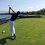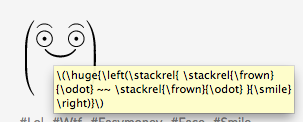# Smile :)

$\huge{\left(\stackrel{ \stackrel{\frown}{\odot} ~~ \stackrel{\frown}{\odot} }{\smile}\right)}$Note by Trevor Arashiro
6 years, 3 months ago

This discussion board is a place to discuss our Daily Challenges and the math and science related to those challenges. Explanations are more than just a solution — they should explain the steps and thinking strategies that you used to obtain the solution. Comments should further the discussion of math and science.

When posting on Brilliant:

• Use the emojis to react to an explanation, whether you're congratulating a job well done , or just really confused .
• Ask specific questions about the challenge or the steps in somebody's explanation. Well-posed questions can add a lot to the discussion, but posting "I don't understand!" doesn't help anyone.
• Try to contribute something new to the discussion, whether it is an extension, generalization or other idea related to the challenge.

MarkdownAppears as
*italics* or _italics_ italics
**bold** or __bold__ bold
- bulleted- list
• bulleted
• list
1. numbered2. list
1. numbered
2. list
Note: you must add a full line of space before and after lists for them to show up correctly
paragraph 1paragraph 2

paragraph 1

paragraph 2

[example link](https://brilliant.org)example link
> This is a quote
This is a quote
    # I indented these lines
# 4 spaces, and now they show
# up as a code block.

print "hello world"
# I indented these lines
# 4 spaces, and now they show
# up as a code block.

print "hello world"
MathAppears as
Remember to wrap math in $$ ... $$ or $ ... $ to ensure proper formatting.
2 \times 3 $2 \times 3$
2^{34} $2^{34}$
a_{i-1} $a_{i-1}$
\frac{2}{3} $\frac{2}{3}$
\sqrt{2} $\sqrt{2}$
\sum_{i=1}^3 $\sum_{i=1}^3$
\sin \theta $\sin \theta$
\boxed{123} $\boxed{123}$

Sort by:

This was too cute. I had to post it. And its 2 in the morning, and I gotta finish an essay :3

$\huge{\left(\stackrel{ \overbrace{\odot ~~ \odot} }{\smile}\right)}$

- 6 years, 3 months ago

What essay, if I may ask? :3

- 6 years, 3 months ago

That is one hell of a "face". :3

- 6 years, 3 months ago

$\begin{Bmatrix} \widetilde { O } & & \widetilde { O } \\ & \quad \int { } & \\ \vdash & \overbrace { \mho }^{ \sqcup } & \dashv \end{Bmatrix}$

- 6 years, 3 months ago

That's cute...

How did you do it?

- 6 years, 3 months ago

\huge{\left(\stackrel{ \overbrace{\odot ~~ \odot} }{\smile}\right)} is the one in my comment

\huge{\left(\stackrel{ \stackrel{\frown}{\odot} ~~ \stackrel{\frown}{\odot} }{\smile}\right)} is the one in my post

ironically note that the smiley face requires \frown :3

- 6 years, 3 months ago

"Where can you find frown in a smiling face?"

"Of course, in Brilliant."

I wish if I could learn some latex. The fonts in latex could be changed, I have seen it on other sites. Don't know if we can change them here or not.

Thanks for code :)

- 6 years, 3 months ago

If you hover over any Latex (as opposed to hyperlinked images), you will be able to see the Latex code. E.g:Staff - 6 years, 3 months ago

I just keep forgetting this feature. Thanks for reminding. This site has some latex fonts. We may not need any font style but still can we have them here?

- 6 years, 3 months ago

$\boxed{\boxed{\boxed{\boxed{\boxed{\boxed{\boxed{\boxed{\boxed{\boxed{\boxed{\boxed{\boxed{\boxed{\boxed{\boxed{\boxed{\boxed{\boxed{\boxed{\boxed{\boxed{\boxed{\boxed{\boxed{\boxed{\boxed{\boxed{\boxed{\boxed{\boxed{\boxed{\boxed{\boxed{\boxed{\boxed{\boxed{\boxed{\boxed{\boxed{\boxed{\boxed{\boxed{\boxed{\boxed{\boxed{\boxed{\boxed{\boxed{\boxed{\boxed{\boxed{\boxed{\boxed{\boxed{\boxed{\boxed{\boxed{\boxed{\boxed{\boxed{\boxed{\boxed{\boxed{\boxed{\boxed{\boxed{\boxed{\boxed{\boxed{\boxed{\boxed{\boxed{\boxed{\boxed{\boxed{\boxed{\boxed{\boxed{\boxed{\boxed{\boxed{\boxed{\boxed{\boxed{\boxed{\boxed{\boxed{\boxed{\boxed{\huge{\left(\stackrel{ \stackrel{\frown}{\odot} ~~ \stackrel{\frown}{\odot} }{\smile}\right)}}}}}}}}}}}}}}}}}}}}}}}}}}}}}}}}}}}}}}}}}}}}}}}}}}}}}}}}}}}}}}}}}}}}}}}}}}}}}}}}}}}}}}}}}}}$

- 5 years, 5 months ago